# JP2005051337A - Image processing method, image processing apparatus, and program - Google Patents

## Info

Publication number
JP2005051337A
JP2005051337A JP2003203461A JP2003203461A JP2005051337A JP 2005051337 A JP2005051337 A JP 2005051337A JP 2003203461 A JP2003203461 A JP 2003203461A JP 2003203461 A JP2003203461 A JP 2003203461A JP 2005051337 A JP2005051337 A JP 2005051337A
Authority
JP
Japan
Prior art keywords
signal
value
pixel
bits
original signal
Prior art date
Legal status (The legal status is an assumption and is not a legal conclusion. Google has not performed a legal analysis and makes no representation as to the accuracy of the status listed.)
Withdrawn
Application number
JP2003203461A
Other languages
Japanese (ja)
Inventor
Masashi Hara

Original Assignee
Fuji Photo Film Co Ltd

Priority date (The priority date is an assumption and is not a legal conclusion. Google has not performed a legal analysis and makes no representation as to the accuracy of the date listed.)
Filing date
Publication date
Application filed by Fuji Photo Film Co Ltd, 富士写真フイルム株式会社 filed Critical Fuji Photo Film Co Ltd
Priority to JP2003203461A priority Critical patent/JP2005051337A/en
Publication of JP2005051337A publication Critical patent/JP2005051337A/en
Application status is Withdrawn legal-status Critical

• 238000003672 processing method Methods 0 title 1
• 230000001976 improved Effects 0 abstract 2
• 230000001965 increased Effects 0 abstract 1
• 230000001629 suppression Effects 0 abstract 1

## Images

•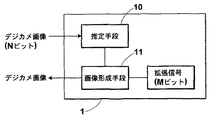••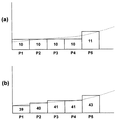••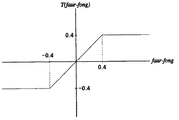•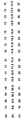••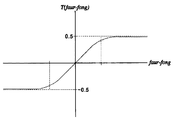•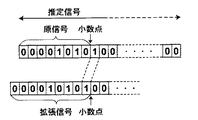•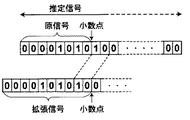•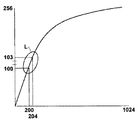•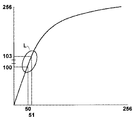## Abstract

<P>PROBLEM TO BE SOLVED: To provide an image processing apparatus capable of suppressing occurrence of a tone jump in a digital image through the improvement of bit resolution so as to prevent an edge part such as a contour from being blurred. <P>SOLUTION: In the case of converting an original signal into an extended signal whose bit resolution is improved more than that of the original signal by increasing n(1) bits to a least significant digit of the original signal resulting from expressing each pixel of the digital image in N(8) bits so as to extend the N(8) bits into M(9) bits, a correction value depending on a difference between the value of the original signal of each pixel and an average value of pixel values of several surrounding pixels of each pixel is added to the original signal to obtain an estimate signal whereby the precision of the value of the original signal of each pixel is improved, the value of the estimate signal is multiplied by a multiple of 2<SP>n</SP>(=a multiple of 2<SP>1</SP>) and thereafter the lower-order digits are rounded off, the estimate signal is converted into the extended signal in higher-order M(9) bits, from which the digital image is formed. In this case, the estimate signal is decided so that the estimate signal returns to the original signal when the lower-order digits of the estimate signal are rounded off. <P>COPYRIGHT: (C)2005,JPO&NCIPI

## Description


BACKGROUND OF THE INVENTION
The present invention relates to an image processing method and apparatus for preventing the occurrence of tone jump in gradation processing of a digital image signal.

[Prior art]
Conventionally, when reproducing a digital image such as an image obtained by photographing with a digital camera or a medical image obtained by photographing with CR or MRI, characteristic variation correction processing by CCD, density conversion, gradation processing The image is processed so that the image of interest can be easily seen.

Of such image processing, in gradation processing, gradation conversion is performed using a look-up table having conversion characteristics from the horizontal axis to the vertical axis as shown in FIG. There is a problem that a tone jump is likely to occur at a portion such as a changing portion L. In particular, when the resolution of the original image on which gradation processing is performed is not sufficient (for example, conversion from 256 gradations to 256 gradations), pixel values that differ by only one gradation in the original image are converted to the third floor after gradation processing. Since the pixel values are different (part L), a point where the color / density suddenly changes appears in the gradation area which should have changed gradually, and the change becomes noticeable. Thereby, it may appear that there is a contour where there is no contour (referred to as a false contour).

Therefore, as a method of reducing the tone jump and false contour generated by increasing the resolution of the digital signal, a random number signal generated by using a random number generation circuit in the least significant digit part (for example, the last two digits) of the digital image signal The effect similar to the case where random numbers are added by using noise on particles of photographic film for data added to the least significant digit part (for example, Patent Document 1) There is something that can be obtained. (For example, Patent Document 2)
Alternatively, a method of reducing tone jumps and false contours by adding up the surrounding pixel values to increase the number of apparent effective bits has been used.

[Patent Document 1]
JP-A-6-203148 
[Patent Document 2]
Japanese Patent Laid-Open No. 10-13673
[Problems to be solved by the invention]
As described above, with the method of adding bits using random numbers or the like, it was possible to make the step due to tone jump difficult to see by blurring uniformly, but the relationship with surrounding pixels was considered. Therefore, even if the resolution is increased in a gradation area where the gradation gradually changes, the gradation cannot be changed along with the change.

On the other hand, in the method of increasing the apparent number of effective bits by adding up the surrounding pixel values, it is possible to change the gradation according to the change where the gradation gradually changes. , Where the entire image is blurred so as to approach the surrounding pixel values, and the pixel values of the original original image change abruptly (for example, where there are edges such as contours) There was a problem that the image was blurred by being influenced by.

In view of this problem, an object of the present invention is to provide an image processing apparatus that can suppress the occurrence of tone jump and does not blur an image more than necessary.

[Means for Solving the Problems]
In the image processing method of the present invention, the number of signal bits is expanded to M (M = N + n) bits by increasing n bits to the least significant digit of the value of the original signal in which each pixel of the digital image is represented by N bits. In an image processing method for converting into an extended signal with improved resolution,
An estimated signal obtained by adding a correction value determined by the difference between the value of the original signal of the pixel and the average value of the pixel values of the neighboring pixels of the pixel to the original signal to increase the accuracy of the value of the original signal of each pixel. Seeking steps,
After rounding the value of the estimated signal by 2 n , rounding the lower digits and converting them to the extended signal of the upper digits M bits to form a digital image,
The correction value is determined to be a value that returns to the value of the original signal when the lower digit of the estimated signal is rounded.

The image processing apparatus of the present invention extends the number of signal bits to M (M = N + n) bits by increasing n bits to the least significant digit of the value of the original signal in which each pixel of the digital image is represented by N bits. In an image processing device that converts an extended signal with improved bit resolution,
An estimated signal obtained by adding a correction value determined by the difference between the value of the original signal of the pixel and the average value of the pixel values of the neighboring pixels of the pixel to the original signal to increase the accuracy of the value of the original signal of each pixel. An estimation means to be obtained;
Image forming means for multiplying the value of the estimated signal by 2 n , rounding the lower digit and converting it to the extended signal of the upper digit M bits to form a digital image;
The correction value is determined to be a value that returns to the value of the original signal when the lower digit of the estimated signal is rounded.

The program of the present invention extends the number of signal bits to M (M = N + n) bits by increasing n bits to the least significant digit of the value of the original signal in which each pixel of the digital image is represented by N bits. In a program for causing a computer to execute image processing for conversion into an extended signal with improved resolution,
An estimated signal obtained by adding a correction value determined by the difference between the value of the original signal of the pixel and the average value of the pixel values of the neighboring pixels of the pixel to the original signal to increase the accuracy of the value of the original signal of each pixel. Seeking steps,
A program for executing a step of rounding a lower digit and converting the value of the estimated signal to 2 n times to convert it into the extended signal of upper digits M bits to form a digital image,
The correction value is determined to be a value that returns to the value of the original signal when the lower digit of the estimated signal is rounded.

“To increase the bit resolution by increasing n bits to the least significant digit of the value of the original signal that represents the pixel in N bits to improve the bit resolution” means that the pixel value of each pixel is By adding the bit of the least significant digit of the bits to be represented, the resolution is improved so that the quantized one pixel value can be represented by a plurality of pixel values in the original gradation. For example, when the pixel value of the image data is expressed by 256 gradations, 8 (N) bits are required. However, when the resolution is increased and the pixel value is expressed by 1024 gradations, 10 (M) bits are required. . Therefore, by increasing 2 (n) bits to 10 bits to the least significant digit of the pixel value represented by 8 bits, the original 8 bits can be divided into four parts that are represented by one pixel value. Expressed in pixel value.

In addition, the “estimated signal in which the accuracy of the value of the original signal is increased” specifically means that the gradation of the original signal is represented by an 8-bit integer, for example, a floating point and the number of significant digits is 8 It is a signal represented by a numerical value that is greater than or equal to a bit. As long as the number of significant digits is 8 bits or more, it may be converted to an integer having a large number of digits even if it is not a floating point.

Also, “determine a value that returns to the value of the original signal when the lower digit of the estimated signal is rounded” is to match the original signal by rounding the lower digit of the estimated signal with higher accuracy by rounding off. The estimation signal is determined, which means that the estimation signal is determined so as to fall within the quantization error range when the original signal is quantized.

“After multiplying the value of the estimated signal by 2n , the lower digit is rounded and converted into an extended signal of M bits of the upper digit”, specifically, for example, the estimated signal calculated in floating point with higher accuracy is 8 bits. When converting to a 10-bit gradation increased by 2 (= n) bits from the gradation, the estimated signal is multiplied by 4 (= 2 2 ) and the lower-order digits after the decimal point are rounded. The value of the signal representing the gray level of this is used as an extended signal.

【The invention's effect】
According to the image processing of the present invention, in order to increase the bit to the least significant digit of the value of the original signal of each pixel of the digital image and improve the bit resolution, the correction value determined by the original signal of the pixel and the neighboring pixels of this pixel In addition to the original signal, a signal value with an increased bit resolution is obtained on the basis of an estimated signal with increased accuracy, so that tone jumps can be suppressed by blurring so as to approach the surrounding pixel values. Furthermore, since the estimated signal with higher accuracy is determined so that the lower digit is rounded, it returns to the original signal value. Therefore, when the pixel value changes suddenly where there is a contour, the original signal is quantized. Since the estimation signal is determined so as not to exceed the error, it is possible to prevent the image from being blurred more than necessary due to the influence of surrounding pixel values.

DETAILED DESCRIPTION OF THE INVENTION
Hereinafter, an embodiment of an image processing apparatus of the present invention will be described with reference to the drawings. FIG. 1 is a block diagram showing the configuration of the present embodiment.

The image processing apparatus 1 expands each pixel of a digital image to M (M = N + n) bits by increasing n bits to the least significant digit of the value of the original signal expressed in N bits, thereby improving the bit resolution. In order to convert the value of the original signal of each pixel to the original signal, a correction value determined by the difference between the value of the original signal of each pixel and the average value of the number of neighboring pixels of the pixel is added to the original signal. An estimation means 10 for obtaining an estimated signal that has been raised, and an image forming means 11 that forms a digital image by rounding the lower digit and converting it to an extended signal of upper M bits after multiplying the value of this estimated signal by 2 n It is provided.

First, the estimation means 10 estimates a signal obtained from an original image before digitization (quantization) from the original signal of the digital image.


Therefore, for example, when a portion where the color / density (image signal) of the image changes slowly as shown by the broken line in FIG. 3 with a gradation with low resolution, the pixel values from P1 to P4 are the same. The pixel value may slightly change in the pixel P5. If such a portion where pixels (P1 to P4) having the same value are arranged is quantized with a gradation having a high bit resolution, the pixel value may gradually change toward the pixel P5. Many. For example, in FIG. 3A, the pixel values from P1 to P4 are “10” and the pixel P5 is “11”. However, as shown in FIG. The pixel value sequentially changes as “39”, “40”, “41”, “41”, “43”.

On the other hand, as shown in FIG. 4, the pixel value may change suddenly between the pixel P4 and the pixel P5. In such a case, the signal obtained from the original image is also likely to be a contour-like edge that suddenly changes as indicated by a broken line. In this way, whether the pixel value changes suddenly or where the pixel value changes slowly as described above, the signal held in the original image is a predetermined range quantized to this pixel value. Only a value within (for example, within the range of the width d in FIG. 2A) can be taken. For example, when the quantized pixel value is “10”, the value forg of the signal obtained from the original image is a quantized value between 9.5 ≦ forg <10.5. That is, it can be said that the quantized pixel value “10” includes such a quantization error.

Considering the above, when estimating the signal that the original image had from the pixel value of the digital image, each pixel is gradually changed toward the pixel value of the neighboring pixels, and A signal that is within the range that can be taken by the original image before quantization (within the range of quantization error) is considered appropriate.

Therefore, the estimated signal estimated to have been in the original image is assumed to have increased accuracy so that the original signal of each pixel of the digital image is brought closer to the pixel value of the neighboring pixel. It can be said that the most appropriate value is determined to return to the value of.

For example, consider a case where the original signal is represented by 8 bits. First, the value of the original signal represented by an 8-bit integer value is converted into a numerical value with a large number of digits such as a floating point, for example, and the original signal value forg of a predetermined pixel and K pixels in the vicinity of this pixel The difference between the average value favr = Σfi / K of the original pixel value fi and the original signal value forg is added to the original signal to obtain an estimated signal with increased accuracy of the original signal value of each pixel. However, if there is a pixel whose pixel value changes suddenly in the neighboring pixels (a pixel whose pixel value suddenly increases or a pixel whose pixel value suddenly decreases), the difference from the average value is calculated. Even if it is added to the original signal as it is, it is affected by the value of its neighboring pixels, and becomes a value exceeding the range of quantization error.

Therefore,
Estimated signal = forg + T (favr−forg) (1)
T: a function determined by the difference from the average value of the pixel values of several neighboring pixels (for example, the function shown in FIG. 5)
And a correction value in the range of 0.5> T (favr-forg)> − 0.5 is added to the original signal so that the estimated signal becomes a value within the quantization error range. That is, when the estimated signal is rounded off, the estimated signal is determined so as to match the value of the original signal.

More specifically, when the difference between the average value of the mask 5 and the original signal is added to the original signal for an image in which pixel values are arranged as shown in FIG. 6A, the original signal is shown in FIG. 6B. The estimated signal is as follows. On the other hand, as shown in FIG. 7A, when there is a place where the pixel value suddenly changes at the arrow, if the difference between the average value of the mask 5 and the original signal is simply added to the original signal, The estimated signal is as shown in FIG. 7B, and the pixels around the arrow are affected by the surrounding pixel values and do not return to the original signal even if rounded off. Therefore, by applying the formula (1), an estimated signal as shown in FIG. 7C is obtained, and the estimated signal can be obtained so as to return to the original signal when the decimal part is rounded off.

The function T shown in the equation (1) may be a sigmoid function as shown in FIG. 8, or any function that can be determined so as to return to the original signal by rounding off after the decimal point.

The image forming unit 11 converts the estimated signal obtained by the estimating unit 10 into an M-bit extended signal in which the resolution of the original signal is increased by n bits, and forms a digital image using the extended signal.

The estimated signal obtained by the estimating means 10 is a value with a large number of digits having an accuracy of N bits or more of the original digital image of the original signal by adding a correction value to the original signal. Therefore, for example, when an 9 (M) -bit extended signal increased by 1 (n) bits to an 8 (N) -bit original signal, the estimated signal is doubled (= 2 1 times) as shown in FIG. ) And rounding off the decimal point, it can be converted into a 9-bit extended signal. For example, if the estimated signal as shown in FIG. 6B is doubled and rounded off, an extended signal as shown in FIG. 6C can be obtained. Similarly, to a 10-bit extension signals with increased 2-bit original signals of 8 bits, then four times the estimated signal as shown in FIG. 10 (= 2 2 times), by rounding off the decimal point For example, it can be converted into a 10-bit extension signal.

Next, the operation of the image processing apparatus 1 will be described. Here, a case where gradation conversion from 256 gradations to 256 gradations is performed using a lookup table will be described.

First, for each pixel of a digital image expressed in 256 gradations, the estimation means 10 adds a correction value determined by the difference from the average value of the pixel values of neighboring pixels to the original signal, and the accuracy of the original signal of each pixel Obtain an estimated signal with

Therefore, the image forming means 11 converts, for example, an estimated signal into a 10-bit extension signal and converts it to 1024 gradations, and then applies a lookup table as shown in FIG. Tone conversion. Comparing the case of converting to the extension signal of FIG. 11 and the case of not converting to the extension signal of FIG. 12, the portion L in FIG. In the portion, three gradations correspond to four gradations. Thus, since the original signal is converted into an extended signal of 1024 gradations and the resolution is increased, the probability that a tone jump occurs even in a place where the gradation changes suddenly as in the L portion. Less.

Even when such processing is performed, the estimated value is estimated within the range of the quantization error, so it is influenced by the values of surrounding pixels where the pixel value changes abruptly as in the contour. The image is never blurred.

As described above, the case where the pixels are arranged in a column in one direction has been described as an example. However, the case where the pixels are arranged two-dimensionally on a plane can be similarly performed.

In the above description, rounding is performed by rounding, but it may be calculated so as to be within the quantization error range when rounded using other methods such as rounding up, rounding down, rounding off, etc. .
[Brief description of the drawings]
FIG. 1 is a block diagram showing a configuration of an image processing apparatus. FIG. 2 is a diagram for explaining gradations to be quantized due to a difference in resolution. FIG. 3 is a diagram for explaining changes in gradations due to a difference in resolution. FIG. 4 is a diagram for explaining a relationship between a change in gradation and a signal value obtained from an original image. FIG. 5 is a diagram showing a function for obtaining an estimated signal (part 1).
FIG. 6 is a diagram for explaining an estimated signal and an extended signal (part 1);
FIG. 7 is a diagram for explaining an estimated signal and an extended signal (part 2);
FIG. 8 is a diagram illustrating a function for obtaining an estimated signal (part 2);
FIG. 9 is a diagram for explaining a method for obtaining an extended signal with a bit resolution increased by 1 bit. FIG. 10 is a diagram for explaining a method for obtaining an extended signal with a bit resolution increased by 2 bits. FIG. 12 is a diagram of a lookup table for gradation conversion from 1024 gradations to 256 gradations using FIG. 12. FIG. 12 is a diagram of a lookup table for gradation conversion from 256 gradations to 256 gradations.
DESCRIPTION OF SYMBOLS 1 Image processing apparatus 10 Estimation means 11 Image formation means

## Claims (3)

1. An extended signal in which the number of signal bits is increased to M (M = N + n) bits to increase the bit resolution by increasing n bits to the least significant digit of the value of the original signal representing each pixel of the digital image in N bits. In the image processing method for conversion,
An estimated signal obtained by adding a correction value determined by the difference between the value of the original signal of the pixel and the average value of the pixel values of the neighboring pixels of the pixel to the original signal to increase the accuracy of the value of the original signal of each pixel. Seeking steps,
After rounding the value of the estimated signal by 2 n , rounding the lower digits and converting them to the extended signal of the upper digits M bits to form a digital image,
The image processing method, wherein the correction value is determined to be a value that returns to the value of the original signal when the lower digit of the estimated signal is rounded.
2. An extended signal in which the number of signal bits is increased to M (M = N + n) bits to increase the bit resolution by increasing n bits to the least significant digit of the value of the original signal representing each pixel of the digital image in N bits. In the image processing device for conversion,
An estimated signal obtained by adding a correction value determined by the difference between the value of the original signal of the pixel and the average value of the pixel values of the neighboring pixels of the pixel to the original signal to increase the accuracy of the value of the original signal of each pixel. An estimation means to be obtained;
Image forming means for multiplying the value of the estimated signal by 2 n , rounding the lower digit and converting it to the extended signal of the upper digit M bits to form a digital image;
The image processing apparatus according to claim 1, wherein the correction value is determined to be a value that returns to a value of an original signal when a lower digit of the estimated signal is rounded.
3. An extended signal in which the number of signal bits is increased to M (M = N + n) bits to increase the bit resolution by increasing n bits to the least significant digit of the value of the original signal representing each pixel of the digital image in N bits. In a program for causing a computer to execute image processing to be converted,
An estimated signal obtained by adding a correction value determined by the difference between the value of the original signal of the pixel and the average value of the pixel values of the neighboring pixels of the pixel to the original signal to increase the accuracy of the value of the original signal of each pixel. Seeking steps,
A program for executing a step of rounding a lower digit and converting the value of the estimated signal to 2 n times to convert it into the extended signal of upper digits M bits to form a digital image,
The program is characterized in that the correction value is determined to be a value that returns to the value of the original signal when the lower digit of the estimated signal is rounded.
JP2003203461A 2003-07-30 2003-07-30 Image processing method, image processing apparatus, and program Withdrawn JP2005051337A (en)

## Priority Applications (1)

Application Number Priority Date Filing Date Title
JP2003203461A JP2005051337A (en) 2003-07-30 2003-07-30 Image processing method, image processing apparatus, and program

## Applications Claiming Priority (1)

Application Number Priority Date Filing Date Title
JP2003203461A JP2005051337A (en) 2003-07-30 2003-07-30 Image processing method, image processing apparatus, and program

## Publications (1)

Publication Number Publication Date
JP2005051337A true JP2005051337A (en) 2005-02-24

# Family

## Family Applications (1)

Application Number Title Priority Date Filing Date
JP2003203461A Withdrawn JP2005051337A (en) 2003-07-30 2003-07-30 Image processing method, image processing apparatus, and program

## Country Status (1)

JP (1) JP2005051337A (en)

## Cited By (4)

* Cited by examiner, † Cited by third party
Publication number Priority date Publication date Assignee Title
JP2009100472A (en) * 2007-10-15 2009-05-07 Intel Corp Bit depth enhancement for scalable video coding
US7649652B2 (en) 2005-10-10 2010-01-19 Samsung Electronics Co., Ltd. Method and apparatus for expanding bit resolution using local information of image
US8204333B2 (en) 2007-10-15 2012-06-19 Intel Corporation Converting video and image signal bit depths
US9171358B2 (en) 2012-09-26 2015-10-27 Olympus Corporation Image editing device and image editing method

## Cited By (6)

* Cited by examiner, † Cited by third party
Publication number Priority date Publication date Assignee Title
US7649652B2 (en) 2005-10-10 2010-01-19 Samsung Electronics Co., Ltd. Method and apparatus for expanding bit resolution using local information of image
JP2009100472A (en) * 2007-10-15 2009-05-07 Intel Corp Bit depth enhancement for scalable video coding
US8204333B2 (en) 2007-10-15 2012-06-19 Intel Corporation Converting video and image signal bit depths
US8208560B2 (en) 2007-10-15 2012-06-26 Intel Corporation Bit depth enhancement for scalable video coding
DE102008051486B4 (en) * 2007-10-15 2017-04-13 Intel Corporation Convert video and video bit depths
US9171358B2 (en) 2012-09-26 2015-10-27 Olympus Corporation Image editing device and image editing method

## Similar Documents

Publication Publication Date Title
JP4544974B2 (en) How to enhance the quality of quantized and decoded images
JP4804660B2 (en) Noise reduction method using color information for digital image processing
US7432985B2 (en) Image processing method
JP2500837B2 (en) Pixel value quantization method
US7149355B2 (en) Image processing apparatus, image processing method, image processing program, and computer-readable record medium storing image processing program
JP4902837B2 (en) How to convert to monochrome image
JP3640979B2 (en) Inverse halftoning method using linear filtering and statistical smoothing
AU635676B2 (en) Image processing apparatus
US20090136152A1 (en) Image correcting apparatus and method, and image correcting program, and look-up table creating apparatus and method, and look-up table creating program
US20080175510A1 (en) Imaging apparatus, noise removing device, noise removing method, program for noise removing method, and recording medium for recording the same
US5200840A (en) Image processing apparatus
JP4565260B2 (en) Signal processing device
US5394250A (en) Image processing capable of handling multi-level image data without deterioration of image quality in highlight areas
JP3921678B2 (en) Image processing method and apparatus
JP2003018403A (en) Image processor
JPH07177351A (en) Method and device for generating halftone pattern for multistage output device
JP3959698B2 (en) Image processing method and apparatus
JP4152313B2 (en) Rendered images using adaptive error diffusion
US6137541A (en) Image processing method and image processing apparatus
US4366507A (en) Shaded picture signal processing system and method
JP4243854B2 (en) Image processing apparatus, image processing method, image processing program, and storage medium
US5208684A (en) Half-tone image processing system
JP2006333431A (en) Image processing method, image processing apparatus, image forming apparatus, computer program, and recording medium
KR20030005262A (en) Screen correcting method and imaging device
JP2010199985A (en) Image processing apparatus, image processing method, and program

## Legal Events

Date Code Title Description
A300 Withdrawal of application because of no request for examination

Free format text: JAPANESE INTERMEDIATE CODE: A300

Effective date: 20061003